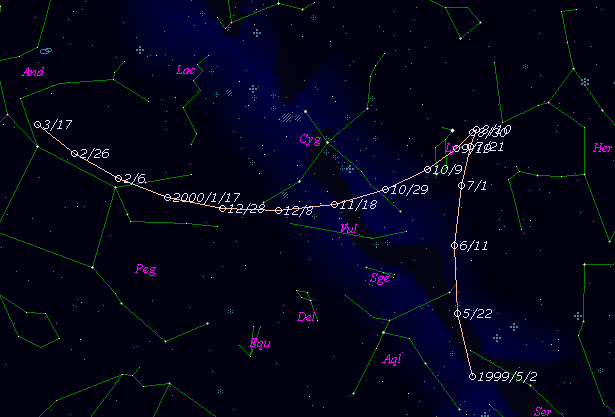\$B%j%K%"WB@1(B

C/1999 K6 ( LINEAR )\$B%W%m%U%#!<%k(B

 \$BH/8+F|(B 1999\$BG/(B 5\$B7n(B20\$BF|(B \$BH/8+8wEY(B 17.6\$BEy(B \$BH/8+ Lincoln Laboratory Near-Earth Asteroid Research project\$B\$B50F;MWAG(B

```   The following improved orbital elements by Kenji Muraoka, are
from 288 observations  1999 May 20  to  Dec. 8, perturbations
by 9 Planets, Moon and 5 minor planets were taken into account.
The mean residual is +/- 0.53 arc seconds.

Epoch  =  1999 Aug. 10.0  TT       JDT = 2451400.5
T  =  1999 July 24.67380       +/- 0.00053 (m.e.) TT
Peri. =   56.76342                +/- 0.00019
Node  =  245.37214                +/- 0.00018   (2000.0)
Incl. =   46.34388                +/- 0.00009
q  =    2.2469770              +/- 0.0000022 AU
e  =    0.9935202              +/- 0.0000032
1/a  =   +0.0028838              +/- 0.0000014 1/AU
( P  =   6457 years )
```\$B@1?^(B\$B8wEYJQ2=(B

```        m1 = 11.0 + 5 log\$B&\$(B + 10.0 log r
```\$B50F;MWAG\$OB<2,7r<#;a\$N7W;;\$K\$h\$k\$b\$N\$G\$9!#(B \$B@1?^\$O(B StellaNavigator Ver.2.0 for Windows (\$B%"%9%H%m%"!<%D(B \$BJTCx(B / \$B%"%9%-!<=PHG6I4)(B) \$B\$G:n@.\$7\$?\$b\$N\$G\$9!#(B \$B8wEY%0%i%U\$O(BComet for Windows\$B\$G:n@.\$7\$?\$b\$N\$G\$9!#(B